# NCERT Solutions For Class 11 Maths Chapter 10 Exercise 10.1

## Chapter 10 Ex.10.1 Question 1

Draw a quadrilateral in the Cartesian plane, whose vertices are $$\left( { - 4,5} \right),\left( {0,7} \right),\left( {5, - 5} \right)$$ and $$\left( { - 4, - 2} \right)$$. Also, find its area.

### Solution

Let $$ABCD$$ be the given quadrilateral with vertices $$A\left( { - 4,5} \right),\;B\left( {0,7} \right),\;C\left( {5, - 5} \right)$$ and $$D\left( { - 4, - 2} \right)$$.

Then, by plotting $$A, B, C$$ and $$D$$ on the Cartesian plane and joining $$AB, \,BC,\, CD$$ and $$DA,$$the given quadrilateral can be drawn as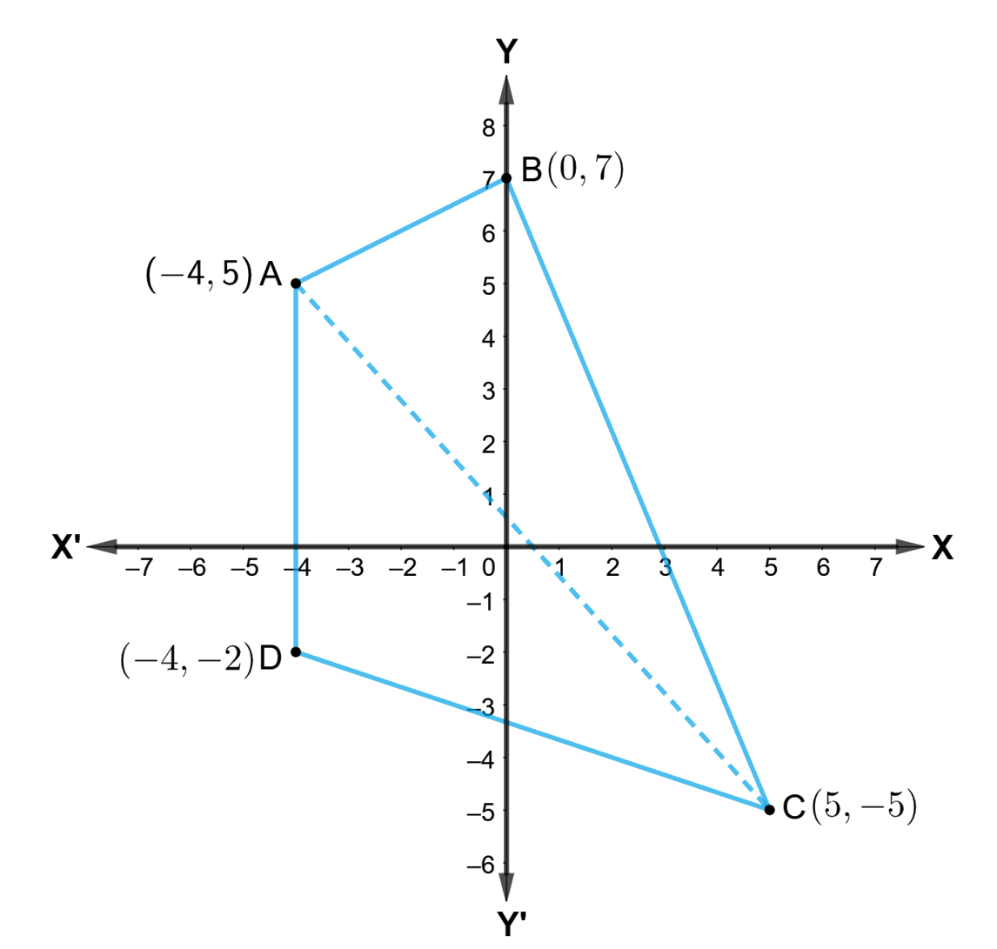To find the area of quadrilateral $$ABCD,$$ we draw one diagonal, say $$AC.$$

Accordingly, $$ar\left( \square ABCD \right)=ar\left( \Delta ABC \right)+ar\left( \Delta ACD \right)$$

We know that the area of a triangles whose vertices are $$\left( {x_1,\,y_1} \right),\left( {{x_2},{y_2}} \right)$$ and $$\left( {{x_3},{y_3}} \right)$$ is

$\frac{1}{2}|{x_1}\left( {{y_2} - {y_3}} \right) + {x_2}\left( {{y_3} - {y_1}} \right) + {x_3}\left( {{y_1} - {y_2}} \right)|$

Therefore,

\begin{align}ar\left( {\Delta ABC} \right) &= \frac{1}{2}\left| { - 4\left( {7 + 5} \right) + 0\left( { - 5 + 5} \right) + 5\left( {5 - 7} \right)} \right|\\&= \frac{1}{2}\left| { - 48 + 0 - 10} \right|\\&= \frac{1}{2}\left| { - 58} \right|\\&= \frac{1}{2} \times 58\\&= 29\end{align}

\begin{align}ar\left( {\Delta ACD} \right) &= \frac{1}{2}\left| {\left( { - 4} \right)\left( { - 5 + 2} \right) + 5\left( { - 2 - 5} \right) + \left( { - 4} \right)\left( {5 + 5} \right)} \right|\\&= \frac{1}{2}\left| {12 - 35 - 40} \right|\\&= \frac{1}{2}\left| { - 63} \right|\\&= \frac{1}{2} \times 63\\&= \frac{{63}}{2}\end{align}

Thus,

\begin{align} ar\left( \square ABCD \right)&=ar\left( \Delta ABC \right)+ar\left( \Delta ACD \right) \\& =\left( 29+\frac{63}{2} \right)sq.\ unit \\& =\left( \frac{58+63}{2} \right)sq.\ unit \\& =\frac{121}{2}sq.\ unit\end{align}

## Chapter 10 Ex.10.1 Question 2

The base of an equilateral triangle with side $$2a$$ lies along the $$y$$-axis such that the midpoint of the base is at the origin. Find vertices of the triangle.

### Solution

Let $$ABC$$ be the given equilateral triangle with side $$2a$$.

Accordingly, $$AB = BC = CA = 2a$$

Assume that base $$BC$$ lies along the $$x$$-axis such that the mid-point of $$BC$$ is at the origin. i.e., $$BO = OC = a$$, where $$O$$ is the origin.

Now, it is clear that the coordinates of point $$C\left( {0,a} \right)$$ , while the coordinates of point $$B\left( {0, - a} \right)$$.

It is known that the line joining a vertex of an equilateral triangle with the mid-point of its opposite side is perpendicular.

Hence, vertex $$A$$ lies on the $$y$$-axis.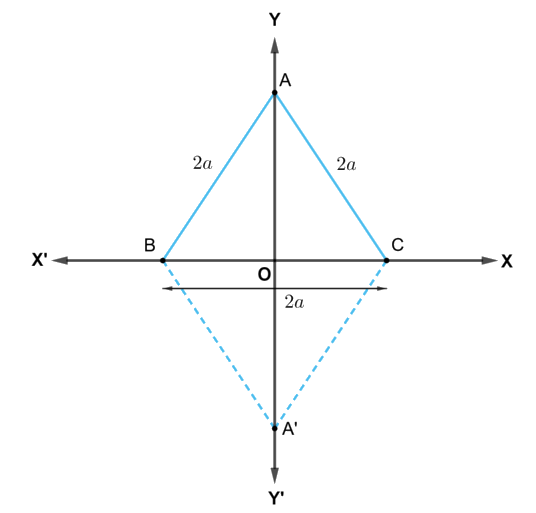On applying Pythagoras theorem to $$\Delta AOC$$, we obtain

\begin{align} A{{C}^{2}}&=O{{A}^{2}}+O{{C}^{2}} \\ O{{A}^{2}}&=A{{C}^{2}}-O{{C}^{2}} \\ & ={{\left( 2a \right)}^{2}}+{{\left( a \right)}^{2}} \\ & =4{{a}^{2}}-{{a}^{2}} \\ & =3{{a}^{2}} \\ OA&=\pm \sqrt{3}a \end{align}

Therefore, coordinates of point $$A\left( { \pm \sqrt 3 a,0} \right)$$

Thus, the vertices of the given equilateral triangle are $$(0,a),\;(0, - a)$$ and $$\left( {\sqrt 3 a,0} \right)$$ or$$(0,a),\;(0, - a)$$ and $$\left( { - \sqrt 3 a,0} \right)$$.

## Chapter 10 Ex.10.1 Question 3

Find the distance between $$P\left( {x_1},{y_1} \right)$$ and $$Q\left( {x_2},{y_2} \right)$$ when: (i) $$PQ$$ is parallel to the $$y$$-axis, (ii) $$PQ$$ is parallel to the $$x$$-axis

### Solution

The given points are $$P\left( {{x_1},{y_1}} \right)$$ and $$Q\left( {{x_2},{y_2}} \right)$$

(i) When $$PQ$$ is parallel to the $$y$$-axis, $${x_1} = {x_2}$$.

In this case, distance between $$P$$ and $$Q= \sqrt {{{\left( {{x_2} - {x_1}} \right)}^2} + {{\left( {{y_2} - {y_1}} \right)}^2}}$$

\begin{align}PQ &= \sqrt {{{\left( {{y_2} - {y_1}} \right)}^2}} \\&= \left| {{y_2} - {y_1}} \right|\end{align}

(ii) When $$PQ$$ is parallel to the $$x$$-axis $${y_1} = {y_2}$$

In this case, distance between $$P$$ and $$Q$$ $$= \sqrt {{{\left( {{x_2} - {x_1}} \right)}^2} + {{\left( {{y_2} - {y_1}} \right)}^2}}$$

\begin{align}PQ &= \sqrt {{{\left( {{x_2} - {x_1}} \right)}^2}} \\&= \left| {{x_2} - {x_1}} \right|\end{align}

## Chapter 10 Ex.10.1 Question 4

Find a point on the $$x$$-axis, which is equidistant from the points $$(7,\, 6)$$ and $$(3, \,4)$$.

### Solution

Let $$(a,\, 0)$$ be the point on the $$X$$- axis that is equidistance from the points $$(7, \,6)$$ and $$(3,\, 4).$$

Accordingly,

\begin{align}&\sqrt {{{\left( {7 - a} \right)}^2} + {{\left( {6 - 0} \right)}^2}} = \sqrt {{{\left( {3 - a} \right)}^2} + {{\left( {4 - 0} \right)}^2}} \\&\Rightarrow \;\sqrt {49 + {a^2} - 14a + 36} = \sqrt {9 + {a^2} - 6a + 16} \\&\Rightarrow \;\sqrt {{a^2} - 14a + 85} = {\sqrt {{a^2} - 6a + 25} ^{}}\end{align}

On squaring on both sides, we obtain

\begin{align}&{a^2} - 14a + 85 = {a^2} - 6a + 25\\&\Rightarrow \;- 14a + 6a = 25 - 85\\&\Rightarrow\; - 8a = 60\\&\Rightarrow\; a = \frac{{60}}{8}\\&\Rightarrow \;a = \frac{{15}}{2}\end{align}

Thus, the required point on the $$x$$-axis is $$\left( {\frac{{15}}{2},0} \right)$$

## Chapter 10 Ex.10.1 Question 5

Find the slope of a line, which passes through the origin, and the mid-point of the segment joining the points $$P\left( {0, - 4} \right)$$ and $$B\left( {8,0} \right)$$.

### Solution

The coordinates of the mid-point of the line segment joining the points

$$P\left( {0, - 4} \right)$$ and $$B\left( {8,0} \right)$$ are $$\left( {\frac{{0 + 8}}{2},\frac{{ - 4 + 0}}{2}} \right) = (4, - 2)$$

It is known that the slope ($$m$$) of a non-vertical line passing through the points $$\left( {x_1},{y_1} \right)$$ and is given by $$m=\frac{{{y}_{2}}-{{y}_{1}}}{{{x}_{2}}-{{x}_{1}}},{{x}_{2}}\ne {{x}_{1}}$$

Therefore, the slope of the line passing through $$\left( 0,0 \right)$$ and $$\left( \text{4},-\text{2} \right)$$ is

$\frac{{ - 2 - 0}}{{4 - 0}} = - \frac{2}{4} = - \frac{1}{2}$

Hence, the required slope of the line is $$- \frac{1}{2}$$

## Chapter 10 Ex.10.1 Question 6

Without using the Pythagoras theorem, show that the points $$\left( 4,4\right),\;\left( 3,5 \right)$$ and $$\left( - 1, - 1 \right)$$ are vertices of a right-angled triangle.

### Solution

The vertices of the given triangles are $$A\left( 4,4 \right),\;B\left( 3,5 \right)$$ and $$C\left( -\text{1},-\text{1} \right)$$.

It is known that the slope ($$m$$) of a non-vertical line passing through the points $$\left( {x_1},{y_1} \right)$$ and $$\left( {x_2},{y_2} \right)$$ is given by $$m=\frac{{{y}_{2}}-{{y}_{1}}}{{{x}_{2}}-{{x}_{1}}},{{x}_{2}}\ne {{x}_{1}}$$

Therefore, slope of AB $$\left( {{m}_{1}} \right)=\frac{5-4}{3-4}=-1$$

Slope of $$BC$$ $$\left( {m_2} \right) = \frac{ - 1 - 5}{ - 1 - 3} = \frac{ - 6}{- 4} = \frac{3}{2}$$

Slope of $$CA$$ $$\left( {{m_3}} \right) = \frac{{4 + 1}}{{4 + 1}} = \frac{5}{5} = 1$$

It is observed that $${m_1}{m_3} = - 1$$

This shows that line segments $$AB$$ and $$CA$$ are perpendicular to each other i.e., the given triangle is right-angled at $$A\left(4,\,4 \right)$$.

Thus, the points $$\left( 4, 4 \right),\;\left( 3, 5 \right)$$ and $$\left( - 1, - 1 \right)$$ are the vertices of a right-angled triangle.

## Chapter 10 Ex.10.1 Question 7

Find the slope of the line, which makes an angle of $$30^\circ$$ with the positive direction of $$y$$-axis measured anticlockwise.

### Solution

If a line makes an angle of $$30^\circ$$ with positive direction of the $$y$$-axis measured anticlockwise, then the angle made by the line with the positive direction of the $$x$$-axis measured anticlockwise is $$90^\circ + 30^\circ = 120^\circ$$.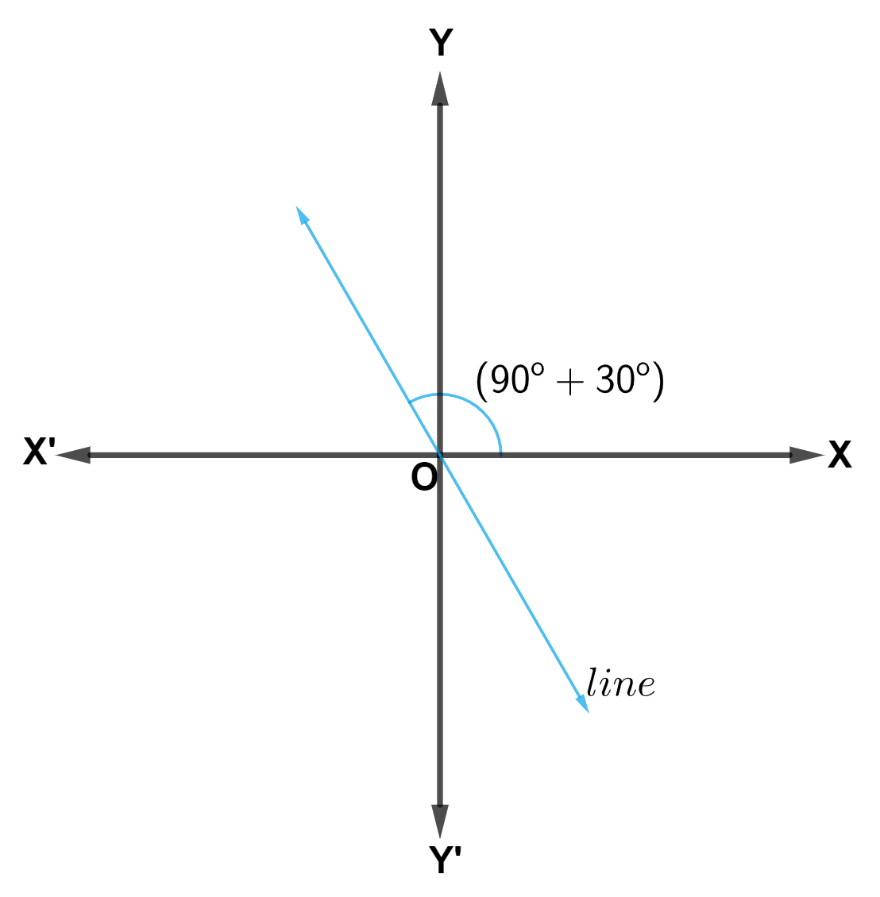Thus, the slope of the given line is

\begin{align} \tan 120{}^\circ &=\text{ }\tan \left( 180{}^\circ -60{}^\circ \right)~ \\& =-\tan 60{}^\circ \\& =-\surd 3\end{align}

## Chapter 10 Ex.10.1 Question 8

Find the value of $$x$$ for which the points $$\left( {x, - 1} \right),\;\left( {2,1} \right)$$ and $$\left(4,\, 5\right)$$ are collinear.

### Solution

If points $$A\left( {x, - 1} \right),\;B\left( {2,1} \right)$$ and $$C\left( 4,5\right)$$ are collinear,

Then, Slope of $$AB =$$ Slope of $$BC$$

\begin{align}&\Rightarrow\; \frac{{1 - \left( { - 1} \right)}}{{2 - x}} = \frac{{5 - 1}}{{4 - 2}}\\&\Rightarrow\; \frac{{1 + 1}}{{2 - x}} = \frac{4}{2}\\&\Rightarrow \;\frac{2}{{2 - x}} = 2\\&\Rightarrow \;2 = 4 - 2x\\&\Rightarrow \;2x = 4 - 2\\&\Rightarrow\; x = 1\end{align}

Thus, the required value of $$x = 1$$

## Chapter 10 Ex.10.1 Question 9

Without using distance formula, show that points $$\left( - 2, - 1\right),\left( {4},0 \right),\left( 3,3 \right)$$ and $$\left( - 3,2\right)$$ are vertices of a parallelogram.

### Solution

Let points $$\left( -2,-1 \right),\left( 4,0 \right),\left( 3,3 \right)$$ and $$\left( -\text{3},\text{ 2} \right)$$ be respectively denoted by $$A,\,B,\, C$$ and $$D.$$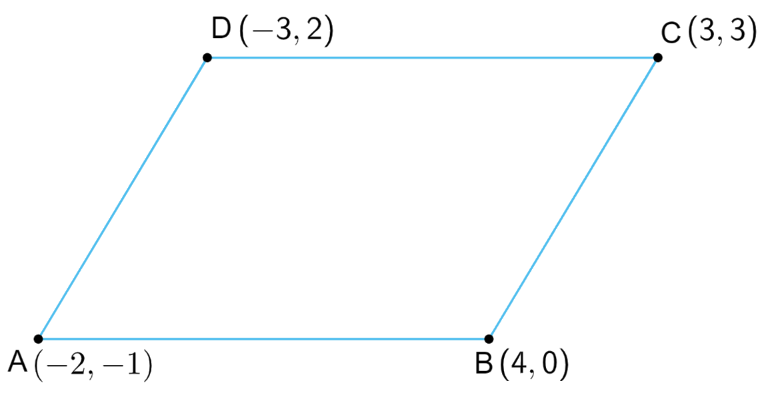Slope of $$AB= \frac{{0 + 1}}{{4 + 2}} = \frac{1}{6}$$

Slope of  $$CD= \frac{{2 - 3}}{{ - 3 - 3}} = \frac{{ - 1}}{{ - 6}} = \frac{1}{6}$$

Therefore, Slope of $$AB =$$ Slope of $$CD$$

Hence, $$AB$$ and $$CD$$ are parallel to each other.

Now,

Slope of $$BC= \frac{3 - 0}{3 - 4} = \frac{3}{ - 1} = - 3$$

Slope of  $$AD= \frac{2 + 1}{ - 3 + 2} = \frac{3}{ - 1} = - 3$$

Therefore, Slope of $$BC =$$Slope of $$AD$$

Hence, $$BC$$ and $$AD$$ are parallel to each other.

Therefore, both pairs of opposite side of quadrilateral $$ABCD$$ are parallel. Hence, $$ABCD$$ is a parallelogram.

Thus, points $$\left(- 2, - 1 \right),\left( 4,0 \right),\left( 3,3 \right)$$ and $$\left( { - 3,2} \right)$$ are the vertices of a parallelogram.

## Chapter 10 Ex.10.1 Question 10

Find the angle between the $$x$$-axis and the line joining the points $$\left(3, - 1 \right)$$ and $$\left( 4, - 2\right)$$

### Solution

The slope of the line joining the points $$\left(3, - 1\right)$$ and $$\left(4, - 2 \right)$$ is

\begin{align}m &= \frac{ - 2 - \left( { - 1} \right)}{4 - 3}\\&= - 2 + 1\\&= - 1\end{align}

Now, the inclination $$\left( {\rm{\theta }} \right)$$ of the line joining the points $$\left(3, - 1 \right)$$ and $$\left(4, - 2 \right)$$ is given by

\begin{align}\tan \theta &= - 1\\\theta &= \left( {90^\circ + 45^\circ } \right)\\\theta & = 135^\circ \end{align}

Thus, the angle between the $$x$$-axis and the line joining the points $$\left( \text{3},-\text{1} \right)$$ and $$\left( \text{4},-\text{2} \right)$$ is $$\text{135}{}^\circ$$.

## Chapter 10 Ex.10.1 Question 11

The slope of a line is double of the slope of another line. If tangent of the angle between them is $$\frac{1}{3}$$, find the slopes of the lines.

### Solution

We know that if $${\rm{\theta }}$$ is the angle between the lines $${l_1}$$ and $${l_2}$$ with slopes $${m_1}$$ and $${m_2}$$ then

$\tan \theta = \left| {\frac{{{m_2} - {m_1}}}{{1 + {m_1}{m_2}}}} \right|$

It is given that the tangent of the angle between the two lines is $$\frac{1}{3}$$ and slope of a line is double of the slope of another line.

Let $$m$$ and $$2m$$ be the slopes of the given lines

Therefore,

\begin{align}\frac{1}{3} &= \left| {\frac{{2m - m}}{{1 + m\left( {2m} \right)}}} \right|\\\frac{1}{3} &= \left| {\frac{m}{{1 + 2{m^2}}}} \right|\\\frac{1}{3} &= \frac{m}{{1 + 2{m^2}}}\end{align}      or      \begin{align} \frac{1}{3} &= \left| {\frac{{m - 2m}}{{1 + m\left( {2m} \right)}}} \right|\\\frac{1}{3} &= \left| {\frac{{ - m}}{{1 + 2{m^2}}}} \right|\\\frac{1}{3} &= \frac{{ - m}}{{1 + 2{m^2}}}\end{align}

Case I

\begin{align}&\frac{1}{3} = \frac{{ - m}}{{1 + 2{m^2}}}\\&1 + 2{m^2} = - 3m\\&2{m^2} + 2m + m + 1 = 0\\&2m\left( {m + 1} \right) + 1\left( {m + 1} \right) = 0\\&\left( {m + 1} \right)\left( {2m + 1} \right) = 0 \end{align}

$$\Rightarrow m = - 1$$ or $$m = - \frac{1}{2}$$

If $$m = - 1$$, then the slopes of the lines are $$-1$$ and $$- 2$$.

If $$m = - \frac{1}{2}$$, then the slopes of the lines are $$- \frac{1}{2}$$ and $$- 1$$.

Case II

\begin{align}&\frac{1}{3} = \frac{m}{{1 + 2{m^2}}}\\&2{m^2} + 1 = 3m\\&2{m^2} - 3m + 1 = 0\\& 2{m^2} - 2m - m + 1 = 0\\&2m\left( {m - 1} \right) - 1\left( {m - 1} \right) = 0\\&\left( {2m - 1} \right)\left( {m - 1} \right) = 0\end{align}

$$\Rightarrow m = 1$$ or $$m = \frac{1}{2}$$

If $$m = 1$$, then the slopes of the lines are $$1$$ and $$2.$$

If $$m = \frac{1}{2}$$, then the slopes of the lines are $$\frac{1}{2}$$ and $$1.$$

Hence, the slopes of the lines are $$-1$$ and $$-2$$, or $$-\frac{1}{2}$$ and $$-\text{1}$$, or $$1$$ and $$2,$$ or $$\frac{1}{2}$$ and $$1.$$

## Chapter 10 Ex.10.1 Question 12

A line passes through $$\left( {{x}_{1}},{{y}_{1}} \right)$$ and $$\left( h,k \right)$$. If slope of the line is $$m$$, show that $$k-{{y}_{1}}=m\left( h-{{x}_{1}} \right)$$

### Solution

The slope of the line passing through $$\left( {{x_1},{y_1}} \right)$$ and $$\left( {h,k} \right)$$ is $$\frac{{k - {y_1}}}{{h - {x_1}}}$$

It is given that the slope of the line is $$m$$

Therefore,

\begin{align}\frac{{k - {y_1}}}{{h - {x_1}}}& = m\\k - {y_1} &= m\left( {h - {x_1}} \right)\end{align}

Hence, $$k - {y_1} = m\left( {h - {x_1}} \right)$$ proved.

## Chapter 10 Ex.10.1 Question 13

If three points $$\left( {h,0} \right),\left( {a,b} \right)$$ and $$\left( {0,k} \right)$$ lie on a line, show that $$\frac{a}{h} + \frac{b}{k} = 1$$

### Solution

If three points $$A\left( {h,0} \right),B\left( {a,b} \right)$$ and $$C\left( {0,k} \right)$$ lie on a line, then

Slope of $$AB =$$ Slope of $$BC$$

\begin{align}\frac{b - 0}{a - h} &= \frac{k - b}{0 - a}\\\frac{b}{a - h} &= \frac{k - b}{{ - a}}\\- ab& = (k - b)(a - h)\\- ab &= ka - kh - ab + bh\\ka + bh &= kh\end{align}

On dividing both sides by $$kh$$, we obtain

\begin{align}\frac{ka}{kh} + \frac{bh}{kh} &= \frac{kh}{kh}\\\frac{a}{h} + \frac{b}{k}& = 1\end{align}

## Chapter 10 Ex.10.1 Question 14

Consider the given population and year graph. Find the slope of the line $$AB$$ and using it, find what will be the population in the year 2010?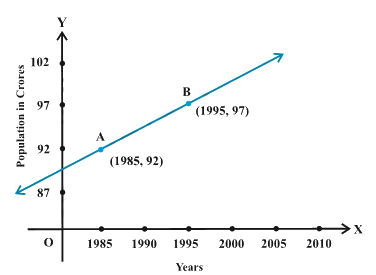### Solution

Since line $$AB$$ passes through points $$A\left( {1985,92} \right)$$ and $$B\left( {1995,97} \right)$$, its slope is

\begin{align} \frac{97-92}{1995-1985}&=\frac{5}{10} \\& =\frac{1}{2}\end{align}

Let $$y$$ be the population in the year 2010.$$A\left( {1985,92} \right)$$

Then, according to the given graph, line $$AB$$ must pass through point $$C\left( {2010,y} \right)$$.

Therefore, Slope of $$AB =$$ Slope of BC

\begin{align}\frac{1}{2} &= \frac{{y - 97}}{{2010 - 1995}}\\\frac{1}{2} &= \frac{{y - 97}}{{15}}\\\frac{{15}}{2} &= y - 97\\y &= 7.5 + 97\\y& = 104.5\end{align}

Thus, the slope of line $$AB$$ is $$\frac{1}{2}$$, while in the year 2010, the population will be $$104.5$$ crores.

Instant doubt clearing with Cuemath Advanced Math Program